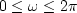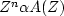# FILTERS IN PARALLEL

We have seen that in a cascade of filters the Z-transform polynomials are multiplied together. For filters in parallel the polynomials add. See Figure 21.

 parallel Figure 21 Filters operating in parallel.We have seen also that a cascade of filters is minimum-phase if, and only if, each element of the product is minimum-phase. Now we will find a condition that is sufficient (but not necessary) for a sum A(Z) + G(Z) to be minimum-phase. First, assume that A(Z) is minimum-phase. Then write(40)
The question as to whether A(Z) + G(Z) is minimum-phase is now reduced to determining whether A(Z) and 1 + G(Z)/A(Z) are both minimum-phase. We have assumed that A(Z) is minimum-phase. Before we ask whether 1 + G(Z)/A(Z) is minimum-phase, we need to be sure that it is causal. Since 1/A(Z) is expandable in positive powers of Z only, then G(Z)/A(Z) is also causal. We will next see that a sufficient condition for 1 + G(Z)/A(Z) to be minimum-phase is that the spectrum of A exceed that of G at all frequencies. In other words, for any real, |A |> |G |. Thus, if we plot the curve of G(Z)/A(Z) in the complex plane, for real,it lies everywhere inside the unit circle. Now, if we add unity, obtaining 1 + G(Z)/A(Z), then the curve will always have a positive real part as in Figure 22.

 garbage Figure 22 A phase trajectory as in Figure 18 left, but more complicated.Since the curve cannot enclose the origin, the phase must be that of a minimum-phase function.

 You can add garbage to a minimum-phase wavelet if you do not add too much.

This abstract theorem has an immediate physical consequence. Suppose a wave characterized by a minimum-phase A(Z) is emitted from a source and detected at a receiver some time later. At a still later time, an echo bounces off a nearby object and is also detected at the receiver. The receiver sees the signal,where n measures the delay from the first arrival to the echo, andrepresents the amplitude attenuation of the echo. To see that Y(Z) is minimum-phase, we note that the magnitude of Zn is unity and the reflection coefficientmust be less than unity (to avoid perpetual motion), so thattakes the role of G(Z). Thus, a minimum-phase wave along with its echo is minimum-phase. We will later consider wave propagation with echoes of echoes ad infinitum.

## EXERCISES:

1. Find two nonminimum-phase wavelets whose sum is minimum-phase.
2. Let A(Z) be a minimum-phase polynomial of degree N. Let.Locate in the complex Z plane the roots of A'(Z). A'(Z) is called maximum phase." (HINT: Work the simple case A(Z) = a0 + a1Z first.)
3. Suppose that A(Z) is maximum-phase and that the degree of G(Z) is less than or equal to the degree of A(Z). Assume |A|>|G|. Show that A(Z) + G(Z) is maximum-phase.
4. Let A(Z) be minimum-phase. Where are the roots ofin the three cases | c | < 1, | c | > 1, | c | = 1? (HINT: The roots of a polynomial are continuous functions of the polynomial coefficients.)